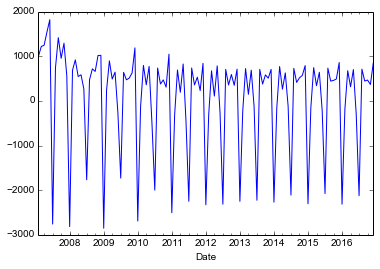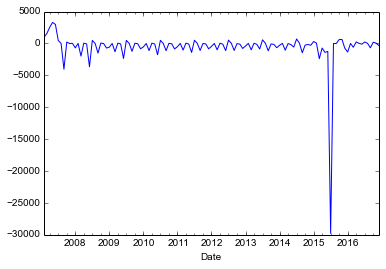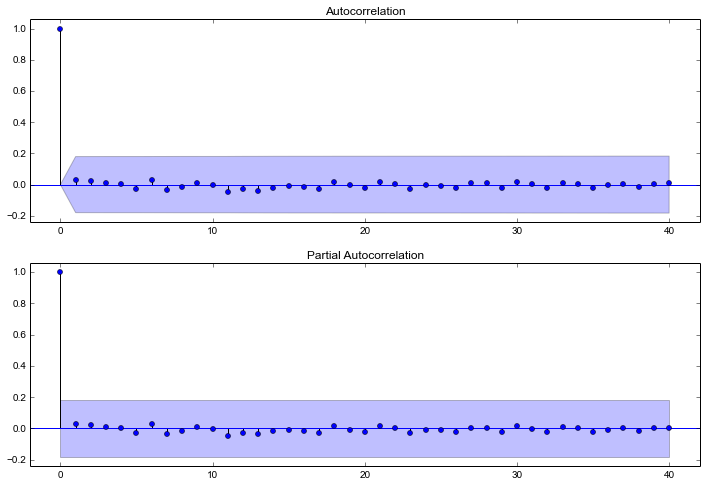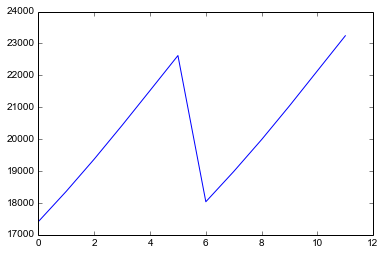Basics of ARIMA Models With Statsmodels in Python

A common problem in many businesses is that of forecasting some value over time. It is useful for setting budgets, understanding sales, and any number of other problems.

In this post, I will go over the basics of a popular forecasting model. Particularly, I will go over ARIMA models.

ARIMA models are univariate models (think sales over time). They are flexible and easily extend to a setting where you have other variables that can influence your target variable (think sales over time driven by marketing spend). In this post, we’ll focus just on the univariate model.

ARIMA stands for Auto-Regressive (AR) Integrated (I) Moving Average (MA). That sounds scary. But it isn’t too bad.

After completing this tutorial you will be able to:

• Develop a Basic ARIMA model using Statsmodels
• Determine if your time series is stationary
• Choose the correct number of AR and MA terms
• Evaluate your model for goodness of fit
• Produce a forecast

Description of Problem

You have a univariate time series that you need to forecast into the future. For concreteness, let’s say that time series is your company’s sales in dollars over time. It is broken down by month for the last ten years. (In reality you are probably the one whipping the data into this shape but we’ll ignore that for now). So you have 120 observations. In fact here is the dataset: salesdata

You want to forecast sales for the next 12 months.

In order to analyze the data. You need to be able to use the data. That is where python’s excellent data manipulation library pandas comes in. Here is some code to get you off to the races.

import pandas as pd pd.read_csv('where/you/saved/the/csv/salesdata.csv') 

Basically, load the pandas library to the python console. Then use pandas to read the csv file. Note that you will need to edit the path to the file to where you saved it. It really is that painless to get the model into python.

At this point it makes a lot of sense to take a look at this data and see what it looks like. Which you can do with the following code:

 df.index=pd.to_datetime(df['Date']) df['Sales'].plot() 

From this code you should get a line chart, that shows how the data looks over time. It looks like this:You can immediately see that this time series is increasing over time, and fairly cyclic. It has two cycles every year, or 1 cycle per 6 months.

What Does An ARIMA Model do?

Okay so at this point you may be asking yourself, why do I use an ARIMA model? It looks like I can do a straight line estimate, and get the right answer, or at the very least a decently close answer. And you very much can. The ARIMA model is all about differencing the series to make it stationary.

It turns out, that with time series when the previous value can affect the current value, you get the wrong standard errors on your coefficients, and you can get the wrong coefficients. OLS is only going to work really well with a stationary time series. An ARIMA model is an attempt to cajole the data into a form where it is stationary.

We do this by taking differences of the variable over time. We have three methods of “taking differences” available to us in an ARIMA model. The AR term, the I term, and the MA term. Let’s actually start with the I term, as it is the easiest to explain. The I term is a full difference. That is today’s value minus yesterday’s value. That’s it.

The AR term is very much related. The way that I like to think of the AR term is that it is a partial difference. The coefficient on the AR term will tell you the percent of a difference you need to take. And the MA term tells you what percent to add back into the error term after differencing. Greene’s book Econometric Analysis, makes the point that this is really about patching up the standard errors. At least that’s what I got out of his discussion of the topic.

At any rate. The point that is germane to the topic is that the way that I view this is in the context of differencing. The whole goal of an ARIMA model is to get the time-series from a non-stationary series to a stationary series. And we are going to apply the terms in such a way that we get there.

Now the problem with this is that there are a lot of ways that we can get to a stationary series by taking full differences, partial differences, and adding partial differences back in. So we need some way to choose which terms to use. In practice, I tend to fit many, many ARIMA models, to see how stable things are. If my coefficients seem relatively robust, I then select the model with the lowest log-likelihood. It isn’t necessarily the best strategy, but in an applied world, I think it makes a lot of sense.

However, the correct way to select the terms that you are going to use is to use an autocorrelation plot and a partial autocorrelation plot to determine the correct number of terms, with an augmented dickey-fuller test.

Taking A Look at Autocorrelations

So the first thing we’re going to do is look at the autocorrelation, and partial autocorrelations for this time series. We can do that by running the following code.

 import statsmodels.api as sm import matplotlib.pyplot as plt fig = plt.figure(figsize=(12,8)) ax1 = fig.add_subplot(211) fig = sm.graphics.tsa.plot_acf(df['Sales'], lags=40, ax=ax1) ax2 = fig.add_subplot(212) fig = sm.graphics.tsa.plot_pacf(df['Sales'], lags=40, ax=ax2) plt.show() 

This code will produces a plot that looks like this:Autocorrelation and Partial Autocorrelation Plot

From the autocorrelation plot we can tell whether or not we need to add MA terms. From the partial autocorrelation plot we know we need to add AR terms. It looks like an AR(1) model might work, but an AR(7) might be appropriate too. But I was a little tricky. I didn’t check to see if the series was stationary yet. You are looking for spikes outside of the shaded confidence intervals.

To check whether or not the series is stationary, we need to do an Augmented Dickey-Fuller Test.  Statsmodels makes this nice.

 print(statsmodels.tsa.stattools.adfuller(x)) 

The null hypothesis is the time series has a unit root. And the results that we get are a test statistic of -1.39 with a p-value of 0.38. Which is well below the 5% threshold so we say that we can’t reject the possibility of a unit root, which means we need to difference the series and then produce our autocorrelation plots. It’s just a minor change to the code.

 fig = plt.figure(figsize=(12,8)) ax1 = fig.add_subplot(211) fig = sm.graphics.tsa.plot_acf(df['Sales'].diff(), lags=40, ax=ax1) ax2 = fig.add_subplot(212) fig = sm.graphics.tsa.plot_pacf(df['Sales'].diff(), lags=40, ax=ax2) plt.show() 

And that gives you this plot that looks like this:Which shows a pattern of MA terms, specifically an MA(6) term looks appropriate. Which makes sense given we have a 6 month cycle. So we’ll give an ARIMA(0,1,6) model a try. What we’ll expect is insignificant MA terms (1-5) and then the 6th will be significant. We’ll then plot the autocorrelations of the residuals, and see what we get along with a q-plot to see if the residuals are normally distributed.

Running the model

Running the ARIMA model described above is fairly easy. Here is the code that you need to run.

 model=sm.tsa.ARIMA(endog=df['Sales'],order=(0,1,6)) results=model.fit() print(results.summary()) 

The first line is where you define the model. The second line you fit the model and save the results. In the last model, you print those results to the screen. Easy right. So let’s take a look at the results.

 ARIMA Model Results ============================================================================== Dep. Variable: D.Sales No. Observations: 119 Model: ARIMA(0, 1, 6) Log Likelihood -1002.613 Method: css-mle S.D. of innovations 1011.299 Date: Thu, 08 Jun 2017 AIC 2021.226 Time: 21:56:07 BIC 2043.459 Sample: 02-01-2007 HQIC 2030.254 - 12-01-2016 ================================================================================= coef std err z P>|z| [95.0% Conf. Int.] --------------------------------------------------------------------------------- const 101.6074 4.979 20.407 0.000 91.849 111.366 ma.L1.D.Sales -0.5296 0.068 -7.826 0.000 -0.662 -0.397 ma.L2.D.Sales -0.2231 0.063 -3.515 0.001 -0.347 -0.099 ma.L3.D.Sales -0.4407 0.078 -5.664 0.000 -0.593 -0.288 ma.L4.D.Sales -0.2231 0.065 -3.411 0.001 -0.351 -0.095 ma.L5.D.Sales -0.5296 0.074 -7.157 0.000 -0.675 -0.385 ma.L6.D.Sales 1.0000 0.058 17.156 0.000 0.886 1.114 Roots ============================================================================= Real Imaginary Modulus Frequency ----------------------------------------------------------------------------- MA.1 -0.8252 -0.5648j 1.0000 -0.4045 MA.2 -0.8252 +0.5648j 1.0000 0.4045 MA.3 0.0941 -0.9956j 1.0000 -0.2350 MA.4 0.0941 +0.9956j 1.0000 0.2350 MA.5 0.9959 -0.0903j 1.0000 -0.0144 MA.6 0.9959 +0.0903j 1.0000 0.0144 ----------------------------------------------------------------------------- 

This model looks pretty good. I happen to know that we could do better, because I constructed the data set. In fact, it’s out of the scope of this tutorial, but you can achieve perfect results using a seasonal ARIMA model. I’ll leave it up to you to figure out how, or maybe a future blog post. For now we’ll just proceed as if this were the correct model, because in the real world you don’t have the luxury of knowing what the correct model is.

One of the things that we are interested in is whether or not the model has acheived the stationarity that we were trying to get. We can check that by looking at the residuals, both overtime, and in an autocorrelation plot. We can do that with the following code:

 results.resid.plot() plt.show() fig = plt.figure(figsize=(12,8)) ax1 = fig.add_subplot(211) fig = sm.graphics.tsa.plot_acf(results.resid, lags=40, ax=ax1) ax2 = fig.add_subplot(212) fig = sm.graphics.tsa.plot_pacf(results.resid, lags=40, ax=ax2) plt.show()

That code will produce these three charts. You’ll notice that the residuals do not appear to be normally distributed and our MA(6) coefficients really haven’t done anything to make our time series more stationary.So maybe that wasn’t the right model. Let’s try fitting an ARIMA(7,1,0) model instead. Maybe that will give us a better fit. Again, remember, the true model requires that we have a seasonal component so we won’t hold our breath, but maybe it will work better.

Here is the relevant code. If you have gone through the rest of the tutorial, this shouldn’t be very surprising code. In fact, I basically copied, pasted, and modified the last 3 code blocks.

 model2=sm.tsa.ARIMA(endog=df['Sales'],order=(7,1,0)) results2=model2.fit() print(results2.summary()) results2.resid.plot() plt.show() fig = plt.figure(figsize=(12,8)) ax1 = fig.add_subplot(211) fig = sm.graphics.tsa.plot_acf(results2.resid, lags=40, ax=ax1) ax2 = fig.add_subplot(212) fig = sm.graphics.tsa.plot_pacf(results2.resid, lags=40, ax=ax2) plt.show() 

And here are the results. A couple of things to note. The covariance matrix is singular. Womp, Womp. This is due to the deterministic way that I generated the data, so don’t read to carefully into this, because the model is over identified (I didn’t add any noise). But also our residuals look a lot better, and there doesn’t appear to be any autocorrelation. Job done as far as that is concerned.

 ARIMA Model Results ============================================================================== Dep. Variable: D.Sales No. Observations: 119 Model: ARIMA(7, 1, 0) Log Likelihood nan Method: css-mle S.D. of innovations nan Date: Thu, 08 Jun 2017 AIC nan Time: 22:31:43 BIC nan Sample: 02-01-2007 HQIC nan - 12-01-2016 ================================================================================= coef std err z P>|z| [95.0% Conf. Int.] --------------------------------------------------------------------------------- const 102.9793 nan nan nan nan nan ar.L1.D.Sales -0.1919 nan nan nan nan nan ar.L2.D.Sales -0.3827 nan nan nan nan nan ar.L3.D.Sales -0.3836 nan nan nan nan nan ar.L4.D.Sales -0.3847 nan nan nan nan nan ar.L5.D.Sales -0.3839 nan nan nan nan nan ar.L6.D.Sales 0.6161 nan nan nan nan nan ar.L7.D.Sales -0.1918 nan nan nan nan nan Roots ============================================================================= Real Imaginary Modulus Frequency ----------------------------------------------------------------------------- AR.1 -1.0000 -0.0000j 1.0000 -0.5000 AR.2 -0.5003 -0.8659j 1.0000 -0.3334 AR.3 -0.5003 +0.8659j 1.0000 0.3334 AR.4 0.5004 -0.8658j 1.0000 -0.1666 AR.5 0.5004 +0.8658j 1.0000 0.1666 AR.6 2.1061 -0.8825j 2.2835 -0.0631 AR.7 2.1061 +0.8825j 2.2835 0.0631 -----------------------------------------------------------------------------Unless you go to a seasonal model, this is probably the best that you are going to be able to do with a simple ARIMA model. So let’s use this model to forecast the next 12 months, and see what you get. To do that you use the forecast method in your results class.

 forecast,std,conf=results2.forecast(12) plt.plot(forecast) 

You can also print the variable forecast to get the exact numbers. Also note that the confidence interval for the forecast is undefined for this model as noted above. Normally, we would view this as a problem, but today we won’t care. You’ll notice that the forecast for the next 12 months looks very reasonable given the previous data.And that’s it.

Wrapping Up

So we saw first how to load some time-series data into python. We determined that the data was not stationary using the Augmented Dickey-Fuller Test, and used autocorrelation plots to determine the order of the ARIMA model we wanted to estimate.

From there we estimated the ARIMA model, evaluated it’s performance in terms of  normality of the residuals (We could and should have been more rigorous with this, but that’s for another blog post.) We also looked for significant autocorrelation in the residuals of the ARIMA model. That helped us to determine that the model we tried was no good.

We then estimated a competing model, which performed much better. We used this model to make our forecasts.

Here is the full code for this tutorial, and on github:

 import pandas as pd import statsmodels.api as sm import matplotlib.pyplot as plt df=pd.read_csv('salesdata.csv') df.index=pd.to_datetime(df['Date']) df['Sales'].plot() plt.show() fig = plt.figure(figsize=(12,8)) ax1 = fig.add_subplot(211) fig = sm.graphics.tsa.plot_acf(df['Sales'], lags=40, ax=ax1) ax2 = fig.add_subplot(212) fig = sm.graphics.tsa.plot_pacf(df['Sales'], lags=40, ax=ax2) plt.show() print(sm.tsa.stattools.adfuller(df['Sales'])) fig = plt.figure(figsize=(12,8)) ax1 = fig.add_subplot(211) fig = sm.graphics.tsa.plot_acf(df['Sales'].diff().dropna(), lags=40, ax=ax1) ax2 = fig.add_subplot(212) fig = sm.graphics.tsa.plot_pacf(df['Sales'].diff().dropna(), lags=40, ax=ax2) plt.show() model=sm.tsa.ARIMA(endog=df['Sales'],order=(0,1,6)) results=model.fit() print(results.summary()) results.resid.plot() plt.show() fig = plt.figure(figsize=(12,8)) ax1 = fig.add_subplot(211) fig = sm.graphics.tsa.plot_acf(results.resid, lags=40, ax=ax1) ax2 = fig.add_subplot(212) fig = sm.graphics.tsa.plot_pacf(results.resid, lags=40, ax=ax2) plt.show() model2=sm.tsa.ARIMA(endog=df['Sales'],order=(7,1,0)) results2=model2.fit() print(results2.summary()) results2.resid.plot() plt.show() fig = plt.figure(figsize=(12,8)) ax1 = fig.add_subplot(211) fig = sm.graphics.tsa.plot_acf(results2.resid, lags=40, ax=ax1) ax2 = fig.add_subplot(212) fig = sm.graphics.tsa.plot_pacf(results2.resid, lags=40, ax=ax2) plt.show() forecast,std,conf=results2.forecast(12) plt.plot(forecast) print(forecast)